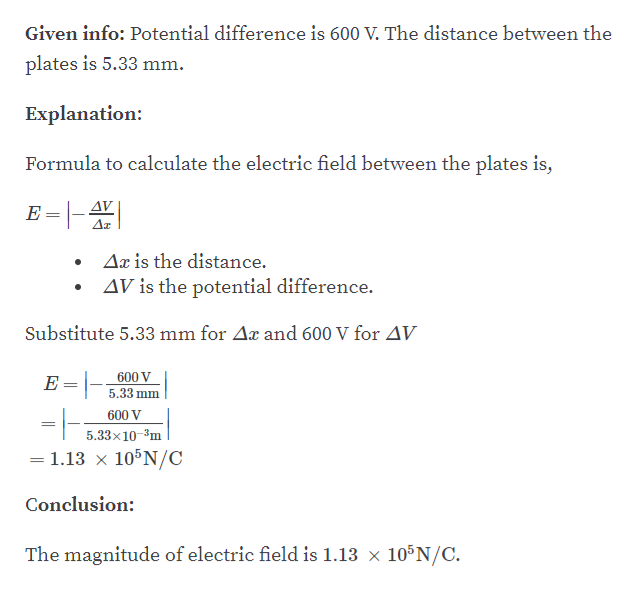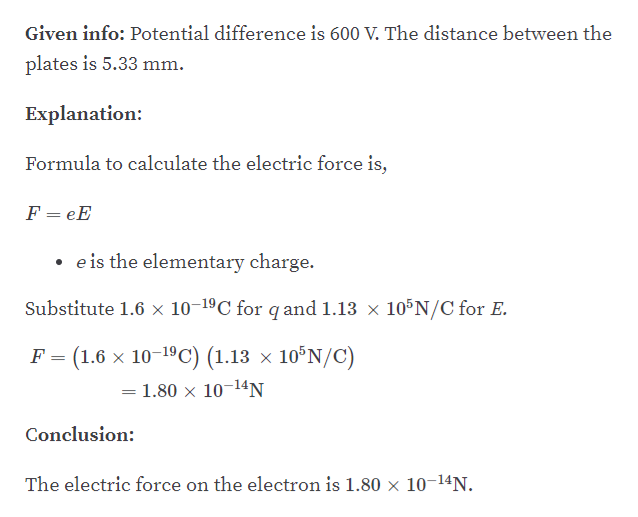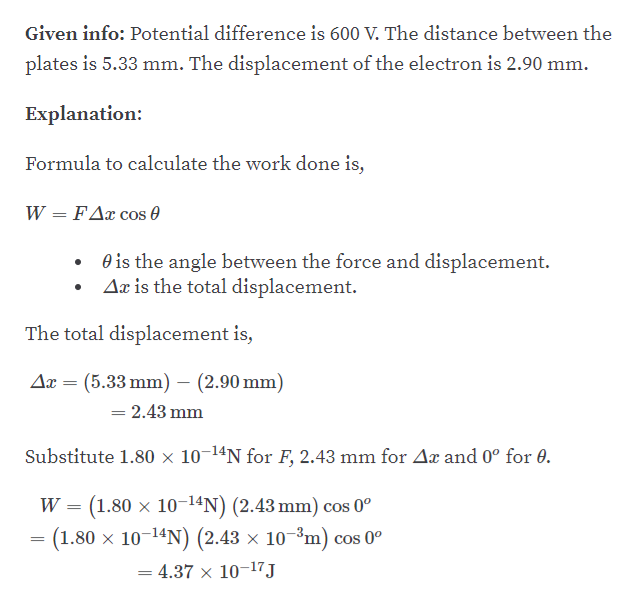# Oppositely charged parallel plates are separated by 5.33 mm. A potential difference of 600. V exists between the plates. (a) What is the magnitude of the electric field between the plates? (b) What is the magnitude of the force on an electron between the plates? (c) How much work must be done on the electron to move it to the negative plate if it is initially positioned 2.90 mm from the positive plate?

Question
266 views

Oppositely charged parallel plates are separated by 5.33 mm. A potential difference of 600. V exists between the plates. (a) What is the magnitude of the electric field between the plates? (b) What is the magnitude of the force on an electron between the plates? (c) How much work must be done on the electron to move it to the negative plate if it is initially positioned 2.90 mm from the positive plate?

check_circle

Step 1

Part A:help_outlineImage TranscriptioncloseGiven info: Potential difference is 600 V. The distance between the plates is 5.33 mm. Explanation: Formula to calculate the electric field between the plates is, E =|-| AV Az Ax is the distance. AV is the potential difference. Substitute 5.33 mm for Ax and 600 V for AV E =|- 600 V 5,33 mm 600 V 5.33x10-³m = 1.13 x 10°N/C %3D Conclusion: The magnitude of electric field is 1.13 x 105N/C. fullscreen
Step 2

Part B:help_outlineImage TranscriptioncloseGiven info: Potential difference is 600 V. The distance between the plates is 5.33 mm. Explanation: Formula to calculate the electric force is, F = eE • e is the elementary charge. Substitute 1.6 x 10-19C for q and 1.13 x 10°N/C for E. F = (1.6 x 10-19C) (1.13 × 10°N/C) = 1.80 x 10¬14N Conclusion: The electric force on the electron is 1.80 x 10-14N. fullscreen
Step 3

Part C:...help_outlineImage TranscriptioncloseGiven info: Potential difference is 600 V. The distance between the plates is 5.33 mm. The displacement of the electron is 2.90 mm. Explanation: Formula to calculate the work done is, W = FAx cos 0 O is the angle between the force and displacement. Aæ is the total displacement. The total displacement is, Ax = (5.33 mm) – (2.90 mm) = 2.43 mm Substitute 1.80 × 10-14N for F, 2.43 mm for Ax and 0° for 0. W = (1.80 x 10-14N) (2.43 mm) cos 0° = (1.80 x 10-14N) (2.43 × 10–³m) cos 0° = 4.37 × 10-17J fullscreen

### Want to see the full answer?

See Solution

#### Want to see this answer and more?

Solutions are written by subject experts who are available 24/7. Questions are typically answered within 1 hour.*

See Solution
*Response times may vary by subject and question.
Tagged in

### Electrostatic Potential and Capacitance